## Power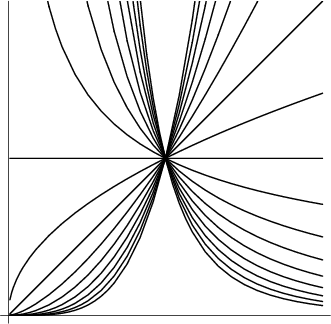The exponent to which a given quantity is raised is known as its Power. The expression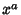is therefore known asto theth Power.'' The rules for combining quantities containing powers are called the Exponent Laws.

Special names given to various powers are listed in the following table.

 Power Name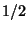Square Root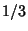Cube Root 2 Squared 3 Cubed

The Sum of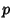th Powers of the firstPositive Integers is given by Faulhaber's Formula,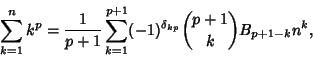where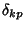is the Kronecker Delta,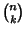is a Binomial Coefficient, and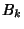is a Bernoulli Number.

Let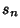be the largest Integer that is not the Sum of distinctth powers of Positive Integers (Guy 1994). The first few values for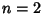, 3, ... are 128, 12758, 5134240, 67898771, ... (Sloane's A001661).

Catalan's Conjecture states that 8 and 9 (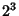and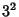) are the only consecutive Powers (excluding 0 and 1), i.e., the only solution to Catalan's Diophantine Problem. This Conjecture has not yet been proved or refuted, although R. Tijdeman has proved that there can be only a finite number of exceptions should the Conjecture not hold. It is also known that 8 and 9 are the only consecutive Cubic and Square Numbers (in either order). Hyyrö and Makowski proved that there do not exist three consecutive Powers (Ribenboim 1996).

Very few numbers of the form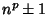are Prime (where composite powers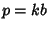need not be considered, since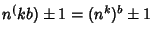). The only Prime Numbers of the form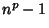for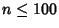and Prime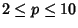correspond to, i.e.,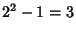,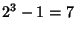,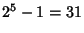, .... The only Prime Numbers of the form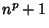forand Primecorrespond to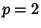with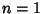, 2, 4, 6, 10, 14, 16, 20, 24, 26, ... (Sloane's A005574).

There are no nontrivial solutions to the equation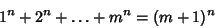for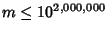(Guy 1994, p. 153).

See also Apocalyptic Number, Biquadratic Number, Catalan's Conjecture, Catalan's Diophantine Problem, Cube Root, Cubed, Cubic Number, Exponent, Exponent Laws, Faulhaber's Formula, Figurate Number, Moessner's Theorem, Narcissistic Number, Power Rule, Square Number, Square Root, Squared, Sum, Waring's Problem

References

Barbeau, E. J. Power Play: A Country Walk through the Magical World of Numbers. Washington, DC: Math. Assoc. Amer., 1997.

Beyer, W. H. Laws of Exponents.'' CRC Standard Mathematical Tables, 28th ed. Boca Raton, FL: CRC Press, p. 158, 1987.

Guy, R. K. Diophantine Equations.'' Ch. D in Unsolved Problems in Number Theory, 2nd ed. New York: Springer-Verlag, pp. 137, 139-198, and 153-154, 1994.

Ribenboim, P. Catalan's Conjecture.'' Amer. Math. Monthly 103, 529-538, 1996.

Sloane, N. J. A. Sequences A001661/M5393 and A005574/M1010 in An On-Line Version of the Encyclopedia of Integer Sequences.'' http://www.research.att.com/~njas/sequences/eisonline.html and Sloane, N. J. A. and Plouffe, S. The Encyclopedia of Integer Sequences. San Diego: Academic Press, 1995.

Spanier, J. and Oldham, K. B. The Integer Powers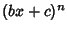and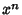'' and The Noninteger Powers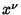.'' Ch. 11 and 13 in An Atlas of Functions. Washington, DC: Hemisphere, pp. 83-90 and 99-106, 1987.